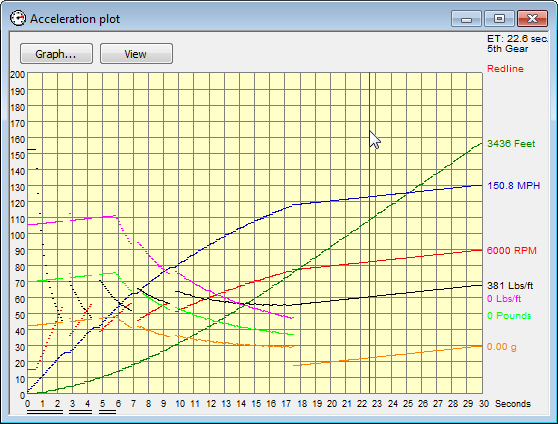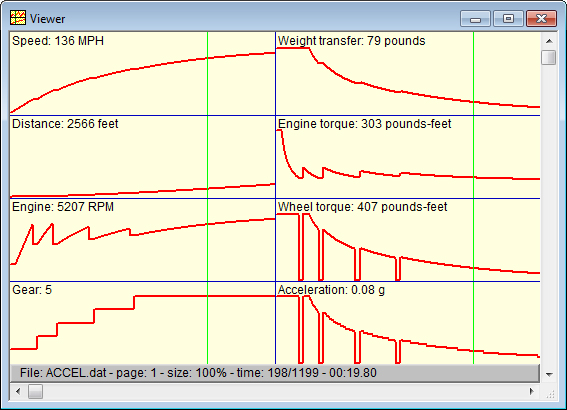## Acceleration Simulation

Speed-Wiz features an advanced acceleration simulation system. The simulator uses an advanced and innovative algorithm that has been tested and perfected over a long period of time. It is also very fast, taking only a small fraction of a second to execute, thanks to some careful code optimization.

The simulation is based on inputs from the acceleration input window, plus the gear ratios and shift points you specify (or calculated shift points based on your specified gear ratios). A wide variety of inputs is available, including shift time, road grade (up or down hill), head wind (or tail wind), and altitude. Of course, settings such as 4-wheel drive and differential type that you specify in the vehicle information section and weather conditions from the conditions input are also used.

Power input can come from specified or calculated maximum power value, maximum torque value, power curve, or torque curve.

The simulator can display its output in numerous formats: acceleration table, acceleration plot, data log view, graphs, and several report views.The acceleration table displays a step-by-step list of the acceleration sequence. The "step size" between each line is fully adjustable and can be based on time, speed, distance, or engine speed.The acceleration plot gives you a graphical representation of the acceleration performance. All of the outputs are plotted, either in real time or in "inspection mode".A view of some selected acceleration parameters in a graph window.Acceleration simulation data can also be sent to the data log viewer where it can be examined yet another way.For a simpler acceleration calculation, see the Dragstrip calculation.

Acceleration rate graph

Acceleration force graph

Acceleration combined graph

Wheel thrust graph